Courses

# Characteristics Of DC Series Motors Electrical Engineering (EE) Notes | EduRev

## Electrical Engineering (EE) : Characteristics Of DC Series Motors Electrical Engineering (EE) Notes | EduRev

The document Characteristics Of DC Series Motors Electrical Engineering (EE) Notes | EduRev is a part of the Electrical Engineering (EE) Course Electrical Machines.
All you need of Electrical Engineering (EE) at this link: Electrical Engineering (EE)

Characteristics of DC Series Motors
(Refer Slide Time: 00:25)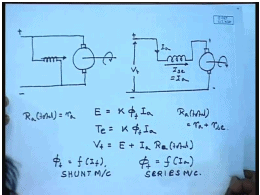So far we have been analysing the performance and characteristics of a DC shunt motor. In a shunt motor the armature is connected across a DC voltage supply, and the field excitation coil is connected across the armature terminal this is the configuration of a shunt machine. Today, we will discuss about the characteristics of a DC series motor, as we know as the name suggests the in a series motor the field winding is connected in series with the armature.

So, while in a shunt motor the field flux remain essentially constant with variation in load torque or armature current in a series motor, it is strongly influenced by the load torque. This brings about a market change in the torque speed characteristics all other characteristics like speed current characteristics of DC series motor which we will discuss today. Whether the excitation is obtained from a shunt field or from a series field the basic equation of a DC machine holds that is the induced voltage E across the brushes is given by back E M F constant into phi the field flux into armature current.

Similarly, the generated torque T e is same K into same field into I a, and of course the terminal equation V t equal to E plus I a into R a total. Now, while for shunt machine phi f is a function of I f which can be controlled independently this is shunt machine. For a series machine, phi f equal to a function of I a, which is given by the magnetization characteristics of the machine, this is series machine. Also R a for shunt machine at least during running condition normally is R a total is normally equal to the simply the armature resistance possibly including the brush drop for a series machine. R a total even during running condition is equal to armature resistance plus the series field winding resistance .

So, let us see how the top speed characteristics and all other characteristics gets modified, because of the dependence of the field flux on armature current. Initially we will assume that the magnetic circuit of a shunt of the machine is linear, that is this f is a linear function and phi f can be given by some flux constant K f into I a. In this case the induced E M F, E becomes K K f; this is omega, omega I a and torque becomes K K f I a square.

(Refer Slide Time: 04:55)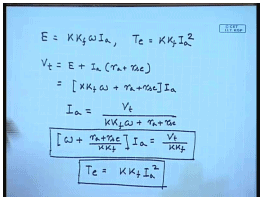So, V t equal to E plus I a r a plus r s c this is equal to K K f omega plus r a plus r s c into I a or I a equal to V t divided by K K f omega plus r a plus r s c or omega plus r a plus r s c by K K f into I a equal to V t by K K f. So, this is the speed versus current characteristics V C, this is like a rectangular hyperbola. Whereas, the torque current characteristics is T e equal to K K f I a square, which is a equation of a parabola. From these two, we can easily derive the torque speed characteristics of the series motor.

(Refer Slide Time: 08:03)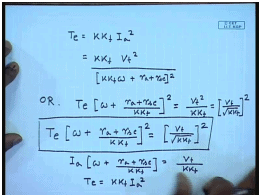That is T e equal to K K f I a square equal to K K f V t square divided by. Or T e into omega plus r a plus r s c divided by, whole square equal to V t square by K K f equal to V t divided by K K f whole square. So, the torque speed characteristics is given by, this is the torque speed characteristics. The current speed characteristics is, torque current characteristics is, let us now draw them.

(Refer Slide Time: 11:09)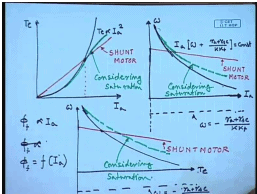Easiest one is to draw the torque current characteristics proportional to I a square. The speed current characteristics and the torque speed characteristics these characteristics asymptotically approaches this line. This line is omega equal to minus, plus r s c by K K f. Again this is somewhat similar, but this line is again omega equal to minus r a plus r s c by K K f. For a comparison let us also draw the corresponding characteristics for shunt motor, for a shunt motor torque is proportional to I a. So, up to some point, this is shunt motor, up to this point shunt motor generates, up to this current shunt motor generates more torque than the series motor, but beyond that series motor can generate a much larger torque.

For the shunt motor these characteristics is a straight line also for a shunt motor torque speed characteristics is a straight line. There are several features to note in a these idealized characteristics of the DC series motor. If we look at the speed current or speed torque characteristics, we find that, the characteristics are asymptotic to I a equal to 0 or T e equal to 0 line; that means, as the load on the motor is reduced the speed tends to increase dangerously. And there is as such no finite no load speed of this series motor therefore, a series motor should never be run on no load then the speed can rise to very high value which can be dangerous. For the same reason it is not possible to operate this motor in a regenerative breaking region, because we know that for regenerating breaking operation the motor must be driven beyond it is no load speed. So, that the current reverses. So, this is not possible for a series motor.

The characteristics we have drawn so far. Are somewhat idealised in the sense, we have assumed that the flux phi f to be proportional to I a while this is true for small values of I a. For larger values of I a, the two effects the saturation of the field and the armature reaction effect tends to violate this condition. So, normally phi f will be a non-linear function of I a and will tend to saturate for larger values of I a. If we take saturation into account then these characteristics gets modified to some extent for example, this torque current characteristics more or less approaches the shunt characteristics for large values of current.

Similarly the dropping speed is not as much, if we consider the saturation dropping torque is also smaller, this is considering saturation. So, when saturation is taken into account, the characteristics of this motor changes in this manner. So, how do we really calculate the characteristic parameters of a series motor taking into account the saturation for that, we will have to first obtain the O C C of the machine.

(Refer Slide Time: 20:05)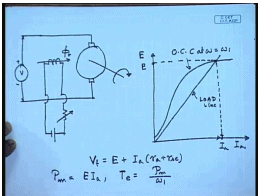For this, the machine will be run as a generator using a prime over at some constants speed and its terminal voltage will be measured. The series field coil will be excited from a separate source of appropriate rating and the data generated can be plotted as shown at omega equal to omega 1. We know that the induced voltage is proportional to the rotational speed therefore; O C C at any other speed can be obtained by simply calling 1 O C C by the ratio of the speed. The other equation the load so called load line is given by V t equal to E plus I a into r a plus r s c, which is again a straight line. At the operating point both the O C C and the load line equation must be satisfied. So, this will be the operating point, this will be the I a and this is E. So, at a given terminal voltage if the machine runs at a speed omega 1 then we can find out the corresponding armature current and the induced voltage in this manner.

Then the mechanical power equal to E I a and the torque developed is thus we will be able to find out the torque at the speed omega 1. Similarly the O C C can be scaled at different speeds and for the same applied voltage, we will be able to find out E and I a and we will be able to generate the torque speed characteristics. So, let us solve one or two problems to explain these methods.

(Refer Slide Time: 23:34)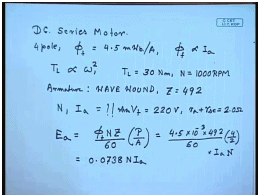Let us say, we have a DC series machine, 4 pole and flux per pole equal to 4.5 milliweber per ampere. We are assuming that phi f is proportional to I a, that is linear magnetization characteristics.

The load torque T L is proportional to omega and omega square and T L equal to 30 Newton meter, when N equal to 1000 R P M. The armature is wave wound as number of conductors then equal to 492. So, we have to determine the speed N and current I a, when this motor is connected to 220 volt, total resistance r a plus r s c equal to 2 ohm. Now, the back E M F as a function of current can be found as follows, number of poles by number of parallel paths, the given data phi f is 4.5 into 10 to the power minus 3 into Z is 492 by 60, P equal to 4 and wave wound therefore A equal to 2 into armature current into R P M. So, E a equal to 0.0738 N I a, this assumes linear magnetisation current.

(Refer Slide Time: 27:39)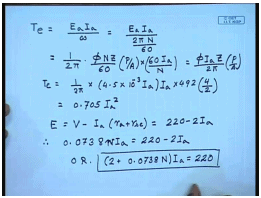And what about torque? Torque we know E a I a by omega equal to E a I a by 2 pi N by 60. This comes to 1 by 2 pi, this is phi N Z by 60 P by A into 60 I a by N this comes to phi I a Z by 2 pi into P by A. So, T e comes to 1 by 2 pi into 4.5into 10 to the power minus 3 into I a within the flux into I a into 492 into number of poles is 4 and number of parallel path is 2. So, this comes to 0.705 I a square, also we know E equal to V minus I a r a plus r s c from given data this comes to 220 minus 2 I a. Therefore 0.0738 N I a equal to 220 minus 2 I a or 2 plus 0.0738 N I a equal to 220, this is the current speed characteristics of this machine. Then torque is 0.705 I a square.

(Refer Slide Time: 31:15)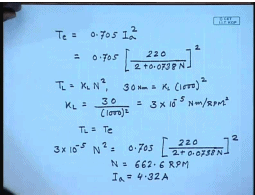Substituting from previous equation, also the load torque is equal to some K L into N square, we have given 30 Newton meter equal to K L into 1000 square. Therefore K L equal to 30 divided by 1000 square which comes to 3 into 10 to the power minus 5 Newton meter per R P M square. Under steady state T L equal to T e therefore, 3 into 10 to the power minus 5 N square equal to 0.705 into 220 divided by 2 plus 0.0738 N whole square. Solving this we get, N equal to 662.6 R P M and the corresponding I a comes to 4.32 amperes.

This is one problem where we have seen, how to find out the speed and current of a DC series machine, when we assume that the magnetic circuit is linear; however, in most cases in most practical cases, the magnetic circuit will not be linear, therefore let us try to solve a problem, where the magnetic circuit of the series motor is not linear.

(Refer Slide Time: 34:14)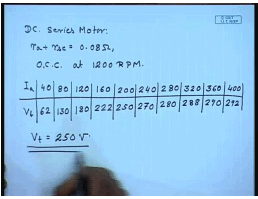So, for that let us assume a DC series motor with the following data. This has r a plus r s c equal to 0.08 ohm. Now, the O C C is determine that 1200 R P M, O C C at 1200 R P M is given by the following data I a, V t at 40 amperes V t is 62 volts, 80 ampere is 130 volts, at 120 amperes that is 180 volts, at 160 amperes this is 222 volt, at 200 amperes it is 250 volts, at 240 amperes it is 270 volts, at 280 amperes it is 280 volts, 292 volts. Suppose this is connected to a voltage source of 250 volts, how I find out the torque speed characteristics of this machine from the given data? For that, let us first ask the question.

(Refer Slide Time: 37:08)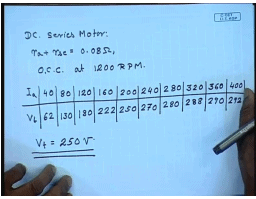Let us some values of armature current I a, let us say equal to 75 amperes, what will be the induced voltage then E should be equal to, we know at 75 amperes; I a equal to 75 amperes and omega equal to N equal to 1200 R P M .

(Refer Slide Time: 37:08)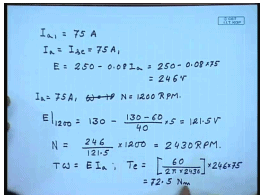We can find out the value from let us say the linear integration of this table. E at 1200 will be equal to E at 80 is 130 minus, is comes to 121.5 volts. So, in order to have a speed of 246, the N must be 246 by 121.5 into 1200. So, this comes to 2430 R P M.

So, when connected to a 250 volt source and drawing a current of 75 ampere the machine will run at 2430 R P M, what will be the torque? T omega equal to E I a, torque will be equal to 60 by 2 pi into 2430 into 246 into 75, this gives to 72.5 Newton meter. Therefore, in this manner, we can calculate for different values of armature current we can find out the torque and speed.

(Refer Slide Time: 41:23)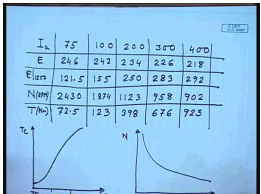So, I a we have done it for 75 amperes, E came out to be 246, E at 1200 from the given magnetisation characteristics is 121.5, N in R P M comes to 2430 and T in Newton meter comes to 72.5. Similarly, we can do the similar calculation for the 100 here it comes to 242, 155, 1874 and 123, 200, 300, for 400 amperes, from this data will be able to draw, let us say torque current characteristics, when connected to a 250 volt supply, speed current characteristics and the torque speed characteristics.

(Refer Slide Time: 44:50)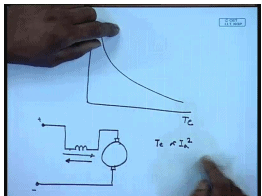We observe that in a DC series machine as the armature current increases the torque increases almost proportional to the square of the armature current which means at large current at low speed at large current. The series machine can generate considerably larger torque compared to a shunt machine, therefore these were preferred for machines where large starting torque is required for example, for traction motors DC series machine has be traditionally used. However, it has to be remembered that a DC series machine does not have intrinsic no load speed. In fact, the load on the DC series machine goes on reducing the speed can increase dangerously.

For example, we have seen here at 400 R P M, where as the speed was 900 R P M at 75 ampere the speed has become almost close to 2500 R P M, which is an increase by a factor of more than 3.5. In a shunt machine this is not so therefore, the speed remains almost constant. So, a DC series machine must be always be run with a load in order to avoid dangerous high speed. A few other salient features about the ideal characteristics of a DC series machine, we see that in a DC series machine the torques speed characteristics is proportional to the terminal voltages square.

In other words it is not possible unlike a shunt motor in a series motor reversing the armature voltage polarity does not produce allow the motor to run in the reverse direction, because when torque is proportional to I a square. Whether current flows in this direction or in this direction, it produces motoring torque only therefore, speed cannot be reversed simply by interchanging the armature terminal. In this cases therefore in most cases for DC series machine, if one wants to generate negative torque then normally it is necessary to reverse the field winding also.

(Refer Slide Time: 49:03)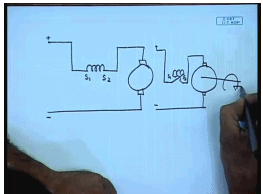That is, in order to generate reverse torque one need to reverse the connection of the series field. That is even with this, it is not possible to obtain degenerative breaking characteristic of the DC series machine as such the reason it is very simple can be understood and follows.

(Refer Slide Time: 50:25)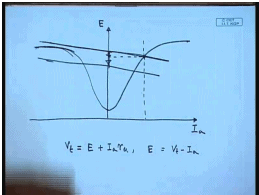For example suppose, we have a DC series machine, whose O C C is given by this curve when operated as a motor, V t equal to E plus I a r a or E equal to V t minus I a therefore, the load line is somewhere here and this is the point where machine operates. Now, suppose I had interchange the polarity of the filter in that case for negative current similar characteristics will be obtained.

However there will be no normally no intersection point between this load line and the reverse magnetisation characteristic E power saturation therefore, even with reverse field winding it will fail to operate in a degenerating breaking mode. The only way degenerating breaking mode is possible is, if by some means the load line can be during breaking operation can be reduced. As we have seen we will see again that this can be achieved by using a electronic power supply called the chopper. Hence a normally a DC series machine will not allow degenerating breaking, but using chopper. You can go for degenerating breaking of even DC series motor. This and other starting and breaking techniques for, and speed control techniques or DC series machine we will discuss in next few classes.

Thank you.

Offer running on EduRev: Apply code STAYHOME200 to get INR 200 off on our premium plan EduRev Infinity!

61 docs|20 tests

,

,

,

,

,

,

,

,

,

,

,

,

,

,

,

,

,

,

,

,

,

;